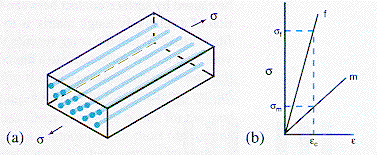Materials and Structure Composite MaterialsComposite materials have their microstructure designed in terms of their macroscopic constituents, e.g. fibers in a homogeneous matrix material. By controlling the choice of fibers, their volume fraction, and alignment, the mechanical properties may be tailored to meet specific design requirements. This is a different level of control than that available for homogeneous materials.The diagram (a) shows a 'uniaxial fiber-reinforced' composite material loaded in the fiber direction. For the continuous fibers shown, the strain is the same in the matrix and the fiber, an isostrain situation. In (b) is shown how the stress on the composite is carried by the fibers and the matrix. Normally, the selected fiber material has a larger Young's modulus than the matrix and for equal elastic strain the fiber stress is higher than the matrix stress. The Young's modulus of the Composite is given by the 'rule of mixtures' i.e. EC = EF VF + EM VM , where VF and VM are the volume fractions of fibers and matrix respectively, also ( VM + VF ) = 1 or VM = (1 - VF ). The elastic modulus along the fiber direction can, therefore, be controlled by selecting the volume fraction of the fibers.From: McMahon and Graham:"The Bicycle and the Walkman," Merion (1992)